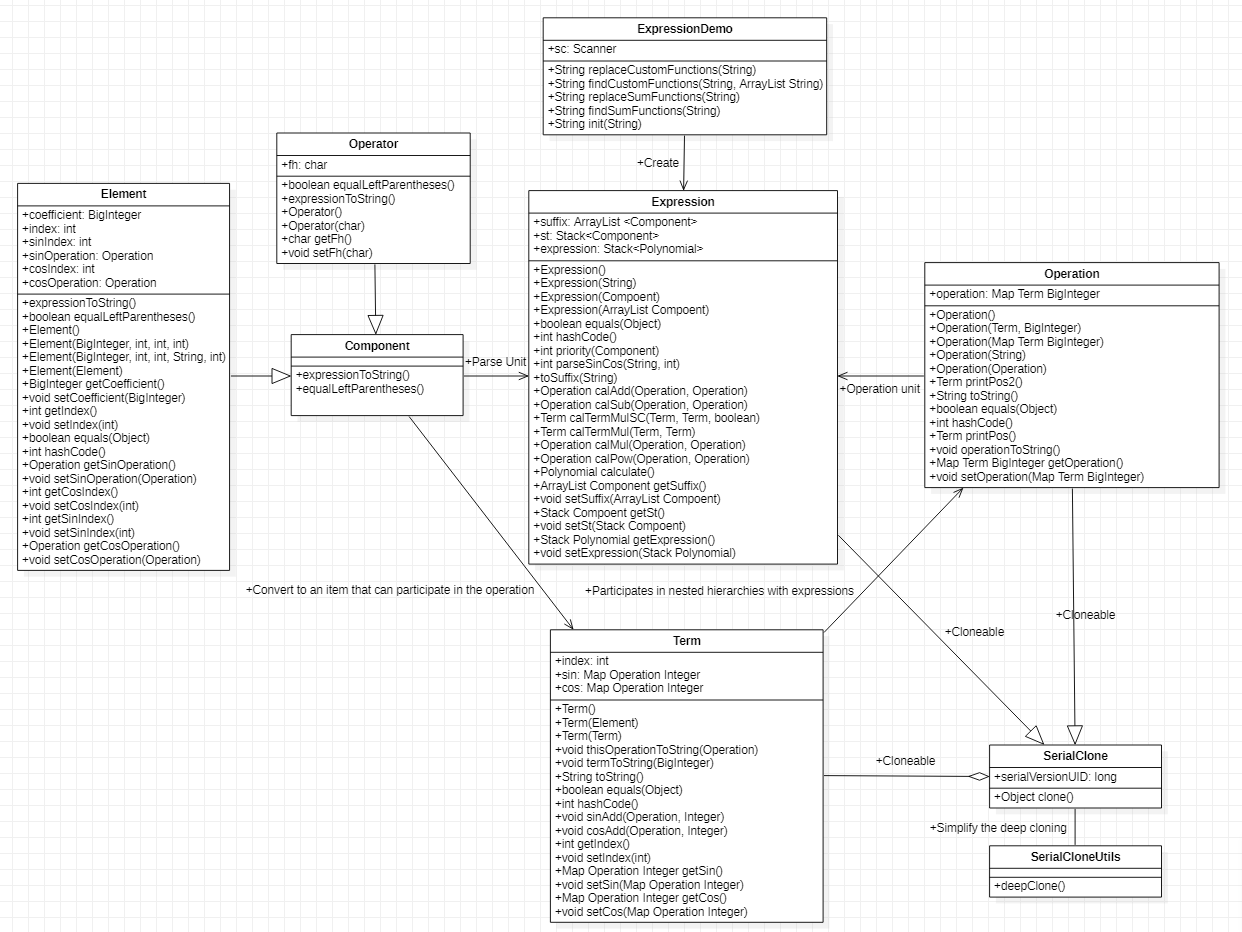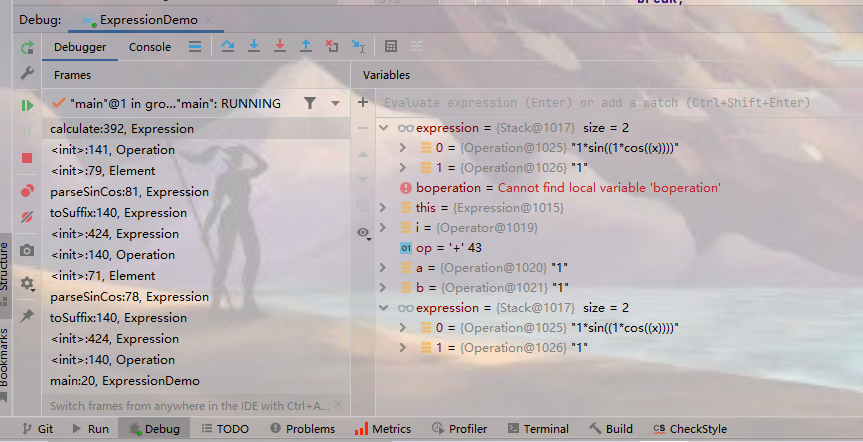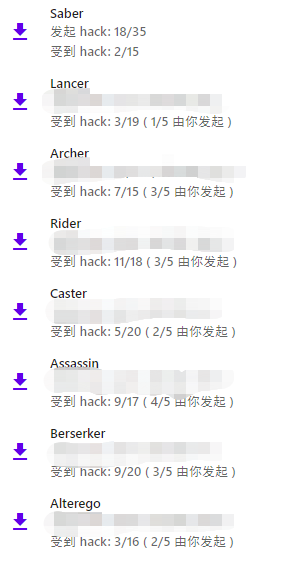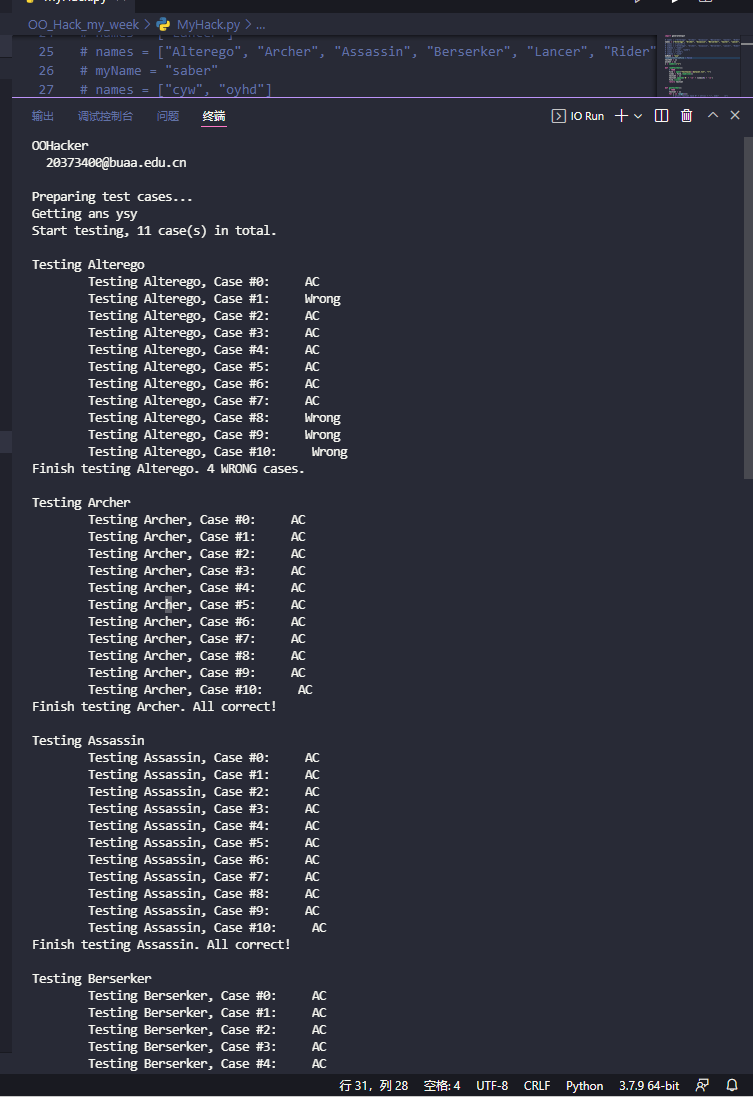# 2022年北航面向对象程序设计第一单元总结

## HW1

### 设计思路

#### UML图### 程序分析

Element.Element() 0.0 1.0 1.0 1.0
Element.Element(BigInteger) 0.0 1.0 1.0 1.0
Element.Element(BigInteger, char, int) 0.0 1.0 1.0 1.0
Element.Element(char, int) 0.0 1.0 1.0 1.0
Element.equalLeftParentheses() 0.0 1.0 1.0 1.0
Element.expressionToString() 0.0 1.0 1.0 1.0
Element.getBy() 0.0 1.0 1.0 1.0
Element.getCoefficient() 0.0 1.0 1.0 1.0
Element.getIndex() 0.0 1.0 1.0 1.0
Element.setBy(char) 0.0 1.0 1.0 1.0
Element.setCoefficient(BigInteger) 0.0 1.0 1.0 1.0
Element.setIndex(int) 0.0 1.0 1.0 1.0
Expression.calAdd(Polynomial, Polynomial) 8.0 1.0 4.0 4.0
Expression.calculate() 8.0 1.0 4.0 8.0
Expression.calMapMul(Map, Map) 7.0 1.0 4.0 4.0
Expression.calMul(Polynomial, Polynomial) 7.0 1.0 4.0 4.0
Expression.calPow(Polynomial, Polynomial) 6.0 3.0 5.0 5.0
Expression.calSub(Polynomial, Polynomial) 8.0 1.0 4.0 4.0
Expression.Expression() 0.0 1.0 1.0 1.0
Expression.Expression(ArrayList) 0.0 1.0 1.0 1.0
Expression.Expression(Component) 0.0 1.0 1.0 1.0
Expression.Expression(String) 0.0 1.0 1.0 1.0
Expression.getExpression() 0.0 1.0 1.0 1.0
Expression.getSt() 0.0 1.0 1.0 1.0
Expression.getSuffix() 0.0 1.0 1.0 1.0
Expression.priority(Component) 2.0 6.0 1.0 6.0
Expression.setExpression(Stack) 0.0 1.0 1.0 1.0
Expression.setSt(Stack) 0.0 1.0 1.0 1.0
Expression.setSuffix(ArrayList) 0.0 1.0 1.0 1.0
Expression.toSuffix(String) 41.0 1.0 23.0 24.0
ExpressionDemo.init(String) 41.0 1.0 23.0 23.0
ExpressionDemo.main(String[]) 0.0 1.0 1.0 1.0
ExpressionDemo.oneJudge(String, int) 2.0 1.0 2.0 2.0
ExpressionDemo.subAccording(String, int, int) 2.0 1.0 2.0 2.0
ExpressionDemo.threeJudge(String, int) 12.0 1.0 8.0 8.0
ExpressionDemo.twoJudge(String, int) 6.0 1.0 4.0 4.0
Operator.equalLeftParentheses() 0.0 1.0 1.0 1.0
Operator.expressionToString() 2.0 1.0 2.0 2.0
Operator.getFh() 0.0 1.0 1.0 1.0
Operator.Operator() 0.0 1.0 1.0 1.0
Operator.Operator(char) 0.0 1.0 1.0 1.0
Operator.setFh(char) 0.0 1.0 1.0 1.0
Polynomial.getPolynomial() 0.0 1.0 1.0 1.0
Polynomial.Polynomial() 0.0 1.0 1.0 1.0
Polynomial.Polynomial(Integer, BigInteger) 0.0 1.0 1.0 1.0
Polynomial.Polynomial(Map) 0.0 1.0 1.0 1.0
Polynomial.polynomialToString() 30.0 6.0 10.0 12.0
Polynomial.printIndex1(BigInteger) 3.0 1.0 3.0 3.0
Polynomial.printIndex2(BigInteger) 3.0 1.0 3.0 3.0
Polynomial.printIndexNormal(Integer, BigInteger) 3.0 1.0 3.0 3.0
Polynomial.printPos() 9.0 3.0 6.0 6.0
Polynomial.setPolynomial(Map) 0.0 1.0 1.0 1.0
Total 230.0 67.0 172.0 185.0
Average 4.34 1.26 3.25 3.49

### bug分析

x**+00


## HW2&&HW3

### 设计思路

#### UML图#### 解析

public static String init(String str, ArrayList<String> func) {
String s = str.replaceAll(" ", "");
s = s.replaceAll("\t", "");
s = findSumFunctions(s);
s = findCustomFunctions(s, func);
s = s.replaceAll(" ", "");
s = s.replaceAll("\t", "");
if (s.charAt(0) == '+' || s.charAt(0) == '-') {
s = '0' + s;
}
s = s.replaceAll("(?<=[^0-9])(0+(?=[0-9]))","");
s = s.replaceAll("^(0+)","");
s = s.replaceAll("\\+{3}","+");
s = s.replaceAll("\\-{3}","-");
s = s.replaceAll("\\+\\-\\-","+");
s = s.replaceAll("\\-\\+\\+","-");
s = s.replaceAll("\\+\\+\\-","-");
s = s.replaceAll("\\-\\-\\+","+");
s = s.replaceAll("\\+\\-\\+","-");
s = s.replaceAll("\\-\\+\\-","+ ");
s = s.replaceAll("\\+\\-","-");
s = s.replaceAll("\\-\\+","-");
s = s.replaceAll("\\+{2}","+");
s = s.replaceAll("\\-{2}","+");
//sin cos
s = s.replaceAll("\\+x","+1*x");
s = s.replaceAll("\\+sin","+1*sin");
s = s.replaceAll("\\+cos","+1*cos");
s = s.replaceAll("\\+\\(","+1*(");
s = s.replaceAll("\\-x","-1*x");
s = s.replaceAll("\\-sin","-1*sin");
s = s.replaceAll("\\-cos","-1*cos");
s = s.replaceAll("\\-\\(","-1*(");
s = s.replaceAll("\\(\\+","(0+");
s = s.replaceAll("\\(\\-","(0-");
s = s.replaceAll("\\*\\*\\+","**");
s = s.replaceAll("\\*\\+","*");
s = s.replaceAll("\\*\\-","*(0-1)*");
s = s.replaceAll("^\\+","");
s = s.replaceAll(" ", "");
s = s.replaceAll("\t", "");
//System.out.println(s);
return s;
}


### 程序分析

Element.Element() 0.0 1.0 1.0 1.0
Element.Element(BigInteger, int, int, int) 0.0 1.0 1.0 1.0
Element.Element(BigInteger, int, int, String, int) 2.0 1.0 1.0 2.0
Element.Element(Element) 0.0 1.0 1.0 1.0
Element.equalLeftParentheses() 0.0 1.0 1.0 1.0
Element.equals(Object) 7.0 4.0 2.0 4.0
Element.expressionToString() 0.0 1.0 1.0 1.0
Element.getCoefficient() 0.0 1.0 1.0 1.0
Element.getCosIndex() 0.0 1.0 1.0 1.0
Element.getCosOperation() 0.0 1.0 1.0 1.0
Element.getIndex() 0.0 1.0 1.0 1.0
Element.getSinIndex() 0.0 1.0 1.0 1.0
Element.getSinOperation() 0.0 1.0 1.0 1.0
Element.hashCode() 0.0 1.0 1.0 1.0
Element.setCoefficient(BigInteger) 0.0 1.0 1.0 1.0
Element.setCosIndex(int) 0.0 1.0 1.0 1.0
Element.setCosOperation(Operation) 0.0 1.0 1.0 1.0
Element.setIndex(int) 0.0 1.0 1.0 1.0
Element.setSinIndex(int) 0.0 1.0 1.0 1.0
Element.setSinOperation(Operation) 0.0 1.0 1.0 1.0
Expression.calAdd(Operation, Operation) 8.0 1.0 4.0 4.0
Expression.calculate() 8.0 1.0 4.0 8.0
Expression.calMul(Operation, Operation) 9.0 1.0 6.0 6.0
Expression.calPow(Operation, Operation) 38.0 10.0 14.0 14.0
Expression.calSub(Operation, Operation) 8.0 1.0 4.0 4.0
Expression.calTermMul(Term, Term) 2.0 1.0 3.0 3.0
Expression.calTermMulSC(Term, Term, boolean) 25.0 4.0 11.0 11.0
Expression.equals(Object) 3.0 4.0 2.0 4.0
Expression.Expression() 0.0 1.0 1.0 1.0
Expression.Expression(ArrayList) 0.0 1.0 1.0 1.0
Expression.Expression(Component) 0.0 1.0 1.0 1.0
Expression.Expression(String) 0.0 1.0 1.0 1.0
Expression.getExpression() 0.0 1.0 1.0 1.0
Expression.getSt() 0.0 1.0 1.0 1.0
Expression.getSuffix() 0.0 1.0 1.0 1.0
Expression.hashCode() 0.0 1.0 1.0 1.0
Expression.parseSinCos(String, int) 18.0 3.0 10.0 12.0
Expression.priority(Component) 2.0 6.0 1.0 6.0
Expression.setExpression(Stack) 0.0 1.0 1.0 1.0
Expression.setSt(Stack) 0.0 1.0 1.0 1.0
Expression.setSuffix(ArrayList) 0.0 1.0 1.0 1.0
Expression.toSuffix(String) 42.0 1.0 26.0 26.0
ExpressionDemo.findCustomFunctions(String, ArrayList) 16.0 5.0 5.0 7.0
ExpressionDemo.findSumFunctions(String) 10.0 4.0 4.0 6.0
ExpressionDemo.init(String, ArrayList) 2.0 1.0 2.0 3.0
ExpressionDemo.main(String[]) 1.0 1.0 2.0 2.0
ExpressionDemo.replaceCustomFunctions(String, String) 4.0 1.0 5.0 5.0
ExpressionDemo.replaceSumFunctions(String) 10.0 2.0 5.0 11.0
Operation.equals(Object) 3.0 3.0 2.0 4.0
Operation.getOperation() 0.0 1.0 1.0 1.0
Operation.hashCode() 0.0 1.0 1.0 1.0
Operation.Operation() 0.0 1.0 1.0 1.0
Operation.Operation(Map) 1.0 1.0 2.0 2.0
Operation.Operation(Operation) 1.0 1.0 2.0 2.0
Operation.Operation(String) 0.0 1.0 1.0 1.0
Operation.Operation(Term, BigInteger) 0.0 1.0 1.0 1.0
Operation.operationToString() 24.0 6.0 7.0 9.0
Operation.printPos() 3.0 3.0 3.0 3.0
Operation.printPos2() 3.0 3.0 3.0 3.0
Operation.setOperation(Map) 0.0 1.0 1.0 1.0
Operation.toString() 28.0 6.0 7.0 11.0
Operator.equalLeftParentheses() 0.0 1.0 1.0 1.0
Operator.expressionToString() 2.0 1.0 2.0 2.0
Operator.getFh() 0.0 1.0 1.0 1.0
Operator.Operator() 0.0 1.0 1.0 1.0
Operator.Operator(char) 0.0 1.0 1.0 1.0
Operator.setFh(char) 0.0 1.0 1.0 1.0
SerialClone.clone() 0.0 1.0 1.0 1.0
SerialCloneUtils.deepClone(T) 1.0 1.0 2.0 2.0
Term.cosAdd(Operation, Integer) 0.0 1.0 1.0 1.0
Term.equals(Object) 4.0 3.0 2.0 6.0
Term.getCos() 0.0 1.0 1.0 1.0
Term.getIndex() 0.0 1.0 1.0 1.0
Term.getSin() 0.0 1.0 1.0 1.0
Term.hashCode() 0.0 1.0 1.0 1.0
Term.setCos(Map) 0.0 1.0 1.0 1.0
Term.setIndex(int) 0.0 1.0 1.0 1.0
Term.setSin(Map) 0.0 1.0 1.0 1.0
Term.sinAdd(Operation, Integer) 0.0 1.0 1.0 1.0
Term.Term() 0.0 1.0 1.0 1.0
Term.Term(Element) 0.0 1.0 1.0 1.0
Term.Term(Term) 2.0 1.0 3.0 3.0
Term.termToString(BigInteger) 21.0 1.0 14.0 14.0
Term.thisOperationToString(Operation) 4.0 1.0 4.0 4.0
Term.toString() 18.0 1.0 7.0 12.0
Total 330.0 136.0 223.0 267.0
Average 3.88 1.60 2.62 3.14

#### 附：度量分析条目解释

• OC：类的非抽象方法圈复杂度，继承类不计入
• WMC：类的总圈复杂度
• ev(G)：非抽象方法的基本复杂度，用以衡量一个方法的控制流结构缺陷，范围是 [1, v(G)]
• iv(G)：方法的设计复杂度，用以衡量方法控制流与其他方法之间的耦合程度，范围是 [1, v(G)]
• v(G)：非抽象方法的圈复杂度，用以衡量每个方法中不同执行路径的数量

### bug分析

1
f(x,y)=(((sin(cos(x))-(+1-sin(cos(y))))))
(f(f(x,x**1),x**+1)*cos(x)**+01)-(cos(sin((x**2))))-(+098782738)**+03

1
f(x,y)=y-(cos(x)**+3-sin(x)**03)
(f(x**3,f(0,1))-+3*x*sin(x))**2+cos(x)*sum(i,1,5,1)*(+1--cos(x))


0
sum(i,-2,-1,i**+2)

0
sum(i,-01,0,(-i**0))


### 测试思路

class DiyFunct:
···
def getDiyFunct():
···
def generate_#(depth = 0,nest = 0,control = 0):
···
def generate_T(depth = 0,nest = 0,control = 0):
···
def generate_Sum():
···


## 补充方法

### 重写equals和hashcode方法

public boolean equals(Object obj) {
return (this == obj);
}


@override
private boolean equals(HashSet<Element> aa, HashSet<Element> bb) {
HashSet<Element> a = new HashSet<>(aa);
HashSet<Element> b = new HashSet<>(bb);
Iterator<Element> it1 = a.iterator();
Iterator<Element> it2 = b.iterator();
while (it1.hasNext()) {
Element ea = it1.next();
while (it2.hasNext()) {
Element eb = it2.next();
boolean judge = ea.getIndex() == eb.getIndex();
boolean judge1 = ea instanceof X && eb instanceof X;
boolean judge2 = ea instanceof Sin && eb instanceof Sin && ((Sin) ea).getUnfoldOperation() == ((Sin) eb).getUnfoldOperation();
boolean judge3 = ea instanceof Cos && eb instanceof Cos && ((Cos) ea).getUnfoldOperation() == ((Cos) eb).getUnfoldOperation();
if (judge && (judge1 || judge2 || judge3)) {
it1.remove();
it2.remove();
}
}
}
return a.size() == 0 && b.size() == 0;
}


@Override
public boolean equals(Object obj) {
if (this == obj) {
return true;
}
if (obj == null || this.getClass() != obj.getClass()) {
return false;
}
Operation op = (Operation) obj;
return Objects.equals(operation, op.getOperation());
}


### 重写clone方法和序列流

public class Term extends SerialClone {
private int index;
private Map<Operation, Integer> sin = new HashMap<>();
private Map<Operation, Integer> cos = new HashMap<>();
··· ···
@Override
protected Term clone() {
//return (Operation)super.clone();
Term p=null;
try{
p=(Term)super.clone();
p.sin=sin.clone();
p.cos=cos.clone();
}catch(CloneNotSupportedException e){
throw new RuntimeException(e);
//e.printStackTrace();
}
return p;
}
}


import java.io.ByteArrayInputStream;
import java.io.ByteArrayOutputStream;
import java.io.IOException;
import java.io.InputStream;
import java.io.ObjectInputStream;
import java.io.ObjectOutputStream;
import java.io.Serializable;

public class SerialCloneUtils {
/**
* 类名SerialCloneUtils
* 使用ObjectStream序列化实现深克隆
* @return Object obj
*/
public static <T extends Serializable> T deepClone(T t) throws CloneNotSupportedException {
// 保存对象为字节数组
try {
ByteArrayOutputStream bout = new ByteArrayOutputStream();
try (ObjectOutputStream out = new ObjectOutputStream(bout)) {
out.writeObject(t);
}

// 从字节数组中读取克隆对象
try (InputStream bin = new ByteArrayInputStream(bout.toByteArray())) {
ObjectInputStream in = new ObjectInputStream(bin);
}
} catch (IOException | ClassNotFoundException e) {
CloneNotSupportedException cloneNotSupportedException;
cloneNotSupportedException = new CloneNotSupportedException();
e.initCause(cloneNotSupportedException);
throw cloneNotSupportedException;
}
}
}

import java.io.Serializable;
/**
* 类名 SerialClone
* 描述 序列化克隆类，只要继承该类，就可以实现深克隆
*
*/
public class SerialClone implements Cloneable, Serializable {
private static final long serialVersionUID = 5794148504376232369L;

@Override
public Object clone() throws CloneNotSupportedException {
return SerialCloneUtils.deepClone(this);
}
}


### 重写toString方法和调试## Hack策略（打点不必要的码，避免被攻击doge）

# artifacts <dir>
#     |-- xxxx_jar <dir>
#     |      |-- xxxx.jar
#     |      |-- *Result.txt
#     |-- *submit <dir>
#     |      |-- *stdin1.txt
#     |      |-- *stdout1.txt
#     |-- TestCases <dir>
#     |-- *Result.txt
#     |-- Hacker.py <this>


names = ["Alterego", "Archer", "Assassin", "Berserker", "Caster", "Lancer", "Rider"]
myName = "oyhd"
testSet = []
ansSet = []


def checkAns(standardAns, usrAns):
if usrAns == None or usrAns == "":
return False
cmp = None
try:
cmp = simplify(simplify(standardAns) - simplify(usrAns))
except:
return False
finally:
return cmp == 0


def writeUsrRes(student, usrWrongList, usrWrongAns):
file = open(student + "_jar\\Result.txt", "w")
length = len(usrWrongList)
if length == 0:
file.write("All correct!")
else:
file.write(str(length) + " wrong case(s):\n\n")
for i in range(length):
file.write(str(i + 1) + "  Case #" + str(usrWrongList[i]) + ":\n")
file.write("stdin:\n")
file.write(str(testSet[usrWrongList[i]]) + "\n")
file.write("stdout:\n")
file.write(str(usrWrongAns[i]) + "\n")
file.write("ans:\n")
file.write(str(ansSet[usrWrongList[i]]) + "\n\n")
file.close()

def writeTotalRes(totalWrongList, totalWrongSet):
file = open("Result.txt", "w")
nOfP = len(names)
for i in range(nOfP):
file.write(names[i])
if len(totalWrongList[i]) == 0:
file.write(": All correct!\n\n")
else:
file.write(": " + str(len(totalWrongList[i])) + " wrong case(s) in total.\n")
for k in totalWrongList[i]:
file.write(str(k) + "\t")
file.write("\n\n")
file.close()
nOfWA = len(totalWrongSet)
tmp = list(totalWrongSet)
if not os.path.exists("submit"):
os.makedirs("submit")
else:
os.system("del /q submit\\*")
for i in range(nOfWA):
file = open("submit\\" + str(i + 1) +"_stdin.txt", "w")
file.write(str(testSet[tmp[i]]))
file.close()
file = open("submit\\" + str(i + 1) +"_stdout.txt", "w")
file.write(str(ansSet[tmp[i]]))
file.close()0
sum(i,1,0,i)-sum(i,-3,-1,(i**2-((-(i**2)**2)**0)))


## 架构设计体验

### 整体架构

#### sin cos和运算的处理

sin cos还有各个运算单独设计一个类写相应的类方法还是直接用一个类融合，这个我选择的还是后者，第二三次作业我开始的时候是sin cos单独设计类，运算的方法都写在了表达式类中，但后来因为过于繁琐的类型下降判断和Map的KeySet的形式需要Map嵌套以及又因需要KeySet的equals方法而被劝退，特别是第二周那会儿，当时重写equals还不是很熟练，也不会深克隆，于是在这种写法下遇到了不小的困难，每次debug的时候都会追踪到Java的Object类的内定义真的很痛苦，以及感觉以及把助教gg问烦了（x，还是感觉把sin cos 多项式写在同一级更透明，对于项的运算也都是在同一个类内进行操作，而不像刚过提到的Map嵌套对项的乘法运算需要遍历Map，maybe这就意味着要remove 😦

### 进一步的化简

贪心优化
sin(0), cos(0)等常数优化
sin(operation)**(2+p)*cos(operation)**q+sin(operation)**p*cos(operation)**(q+2) （除此以外的其他项的参数相等）
cos(-exp)=cos(exp) (我做了首项为正的规则，这个我本次当做了默认优化)
sin(exp)+sin(-exp)=0
cos(exp)+cos(-exp)=2*cos(exp)
1-sin(exp)**2=cos(exp**2)

sin(exp1)cos(exp2)+cos(exp1)sin(exp2)=sin(exp1+exp2)
cos(exp1)cos(exp2)-sin(exp1)sin(exp2)=cos(exp1+exp2)

sin(exp1+exp2)+sin(exp1-exp2)=2*sin(exp1)*cos(exp2)
2*cos(exp1)*cos(exp2)=cos(exp1+exp2)+cos(exp1-exp2)

2*sin(exp)*cos(exp)=sin(2*exp)
cos(exp)**2-sin(exp)**2=cos(2*exp)
4*cos(exp)**3-3*cos(exp)=cos(3*exp)
-4*sin(exp)**3+3*sin(exp)=sin(3*exp)


（所以我认为把字符串留下然后一次一次地运算、试错化简还是很有必要的，毕竟运算速度上在本次作业根本不算什么困难，第一次只需要在乘法的时候把x的乘法项指数相加、常数的加减做了程序的运行就已经非常快了，实测一般只需要0.4秒左右，而评测机支持10秒，并且后续的多次试错化简的速度一定会比第一次快很多，所以我觉得我这种试错化简很有意义）

## 心得体会posted @ 2022-03-25 21:28  Oh_so_many_sheep  阅读(433)  评论(0编辑  收藏  举报